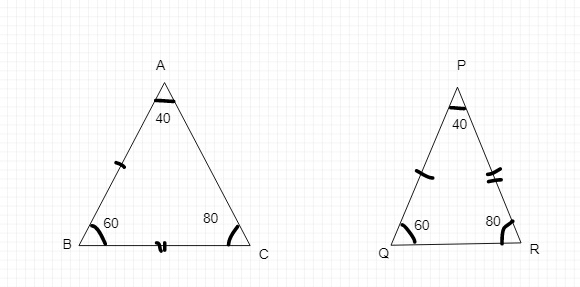# Draw a rough sketch of two triangles such that they have five pairs of congruent parts but still the triangles are not congruent.In the above figures,

∠A = ∠P,

∠B = ∠Q and ∠C = ∠R

AB = PQ and BC = PR

But,

AC ≠ QR

Therefore, 5 parts of the triangles are equal but still the triangles are not congruent.

Updated on: 10-Oct-2022

35 Views